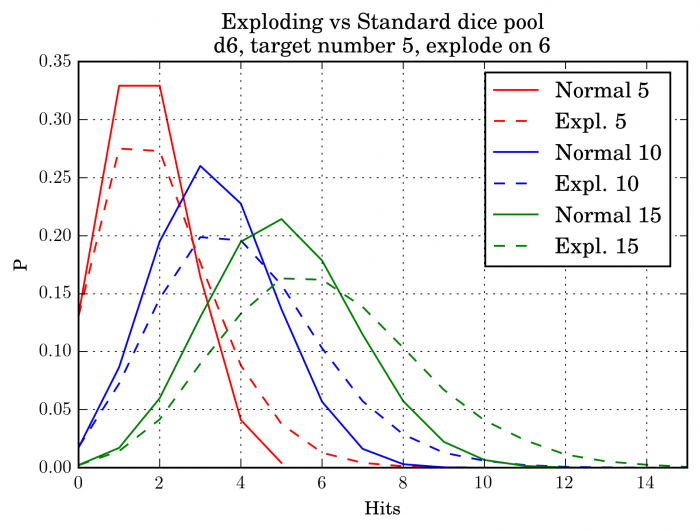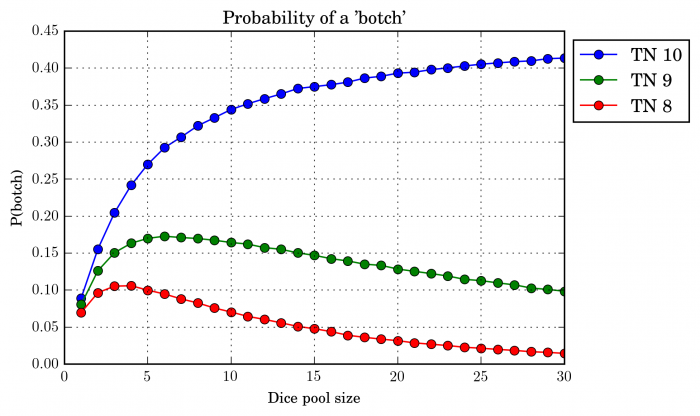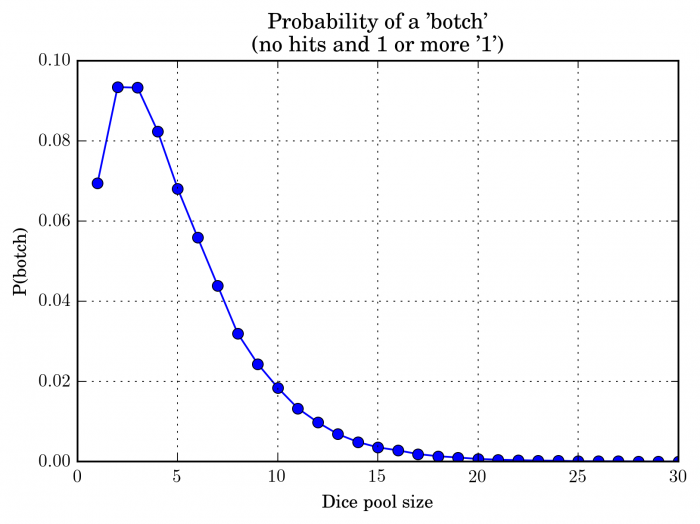In the last post, I mentioned a few examples of common modifications to the “pure” dice pool mechanic. I’ll cover a couple of them here.

#### Exploding dice

The fist case is the so called exploding dice, i.e. if a die comes up with the (usually) maximum possible result, then another die is rolled and added to the dice pool. Eg. a player rolls 5 d6s, and gets 1,1,3,6,6. The player then rolls two more d6s, say 2,6. The second six again adds another die which gives 2. Given the target number of 5, the final result is then 3 hits. So how does this affect the probability distribution? Well, I’m glad that you asked! First consider the expected number of hits for each die. With standard dicepool, the probability of getting a hit is p. For the exploding dice, we need to account for the possibility of getting another roll. Let’s call $p_{max}$ the probability of exploding. So first there is a chance of getting a hit. Then there is a  $p_{max}$ chance of getting another roll that gives a hit with probability of p and again there is a probability $p_{max}$ of the additional die exploding and giving another roll with probability p of getting a hit and so on. This can be expressed as $$p + p_{max}\left(p + p_{max}(p + p_{max}(\ldots))\right)$$ This can be expanded as$$p +p \cdot p_{max} + p \cdot p_{max}^{2} + p \cdot p_{max}^{3} + \ldots$$

which is a converging geometric series. Expressing it as a sum, we get $$\sum_{i=0}^{\infty}p p_{max}^i = \frac{p}{1-p_{max}}.$$ Plugging in some numbers, let’s set p=1/3 and $p_{max}$ = 1/6, we get  E(hits) = 2/5$\approx 0.4$. The difference between exploding dice pool and a standard one is illustrated in the following figure.Note that (obviously) exploding dice allow values that are impossible for the standard dice pool, e.g. about 1% of time an exploding dice pool of 5 dice nets 6 hits. The expected value does not shift much, but there is a noticeable shift and widening of the distribution towards higher values.

#### Critical failure

Some systems have implemented a critical failure condition. For example, in early World of Darkness system, a critical failure (or “botch”) happened when the result of the dice pool contained more 1s than hits. In the original storyteller system, the target number was not constant. Let’s see how the system performs for a few different target numbers. The results are shown in the next figure.There you see the infamous increase in botching with increasing dice pool size for very difficult tasks meaning of course that the more competent the character is, the more likely the character is to hilariously fail instead of just failing. The probability of botching goes over 30% very quickly and even for easier tasks, you should expect to see quite a few botches.

In later editions, a botch only happened if  a roll had no hits and at least one “1”. In this version of the system, the target number was also fixed at 8. In this situation, botches are much less likely.Once you reach 10 die pool, you have only about 2% chance of getting a critical failure on any test.

I originally thought I’d include the consecutive dice pools here, but their treatment is much more involved than the scope of this post. However, the mean is very easy to compute. The first dice pool gets you on average $k_1 = p_1 n$ hits and the second $k_2 = p_2 k_1 = p_2 p_1 n$. So for example, in Warhammer, let’s say 20 goblins hit on 5s and wound on 4s, you would expect $\frac{1}{2}\frac{1}{3} 20 = \frac{20}{6} \approx 3.33$ wounds inflicted on the enemy.

## 2 Replies to “Dice pools – Addendum”

1.Jonathan Dupuis says:

Hi,

Awesome work, very interesting outline of how to evaluate the probabilities and distribution of dice pool.

I noticed that you discuss the mathematics of exploding values on expected mean result, and while you show the effect on the distribution you don’t elaborate on how to generate the exploding dice distribution. How did you proceed? I am currently trying to evaluate the effect of exploding dice pool for World of Darkness and their 10, 9 and 8-again are giving me nightmares!

Thanks!

1.sampos says:

Hi,

Thanks! To be honest, this was quite some time ago so I might not remember all details of my thinking at the time. I think that in the case of the distributions, I just used a simple Monte Carlo -generation where I simulated each die with logic to account for exploding on certain results. I’m not sure if it is worth it to calculate the analytic formula for the distribution. Even for game design, the number of cases (opposed etc.) should be manageable to just do with simulations (which should be fast on any modern-ish computer).

Maybe this should be another post, simulating various systems in general.